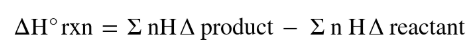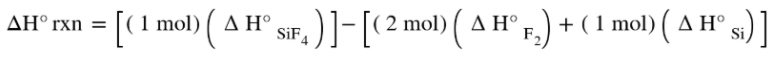# Problem: Using the data in Appendix G, calculate the standard enthalpy change for the following reaction:(a) Si(s) + 2F2(g) ⟶ SiF4(g) ΔH°SiF4 = -1615 kJ/mol

###### FREE Expert Solution
• ΔH°rxn can be solved by getting the difference of ΣΔHproducts and ΣΔHreactions. Equation will appear as• Recall that the standard molar enthalpies for elements in their standard form (pure form in natural conditions) will always be 0.
80% (495 ratings)###### Problem Details

Using the data in Appendix G, calculate the standard enthalpy change for the following reaction:

(a) Si(s) + 2F2(g) ⟶ SiF4(g)

ΔH°SiF= -1615 kJ/mol